L1正则化

L2正则化

弹性网络

# 普通最小二乘法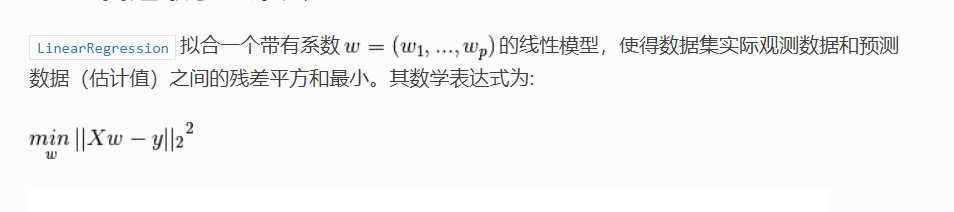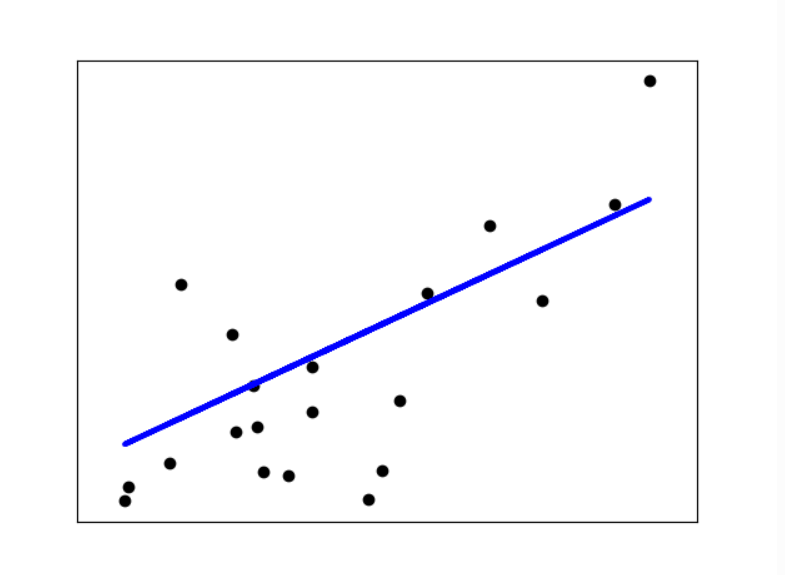# 范数

1-范数：║x║1=│x1│+│x2│+…+│xn│

2-范数：║x║2=（│x1│2+│x2│2+…+│xn│2）1/2

∞-范数：║x║∞=max（│x1│，│x2│，…，│xn│）

|<x,y>| = ||xH*y| ≤ ║x║p║y║q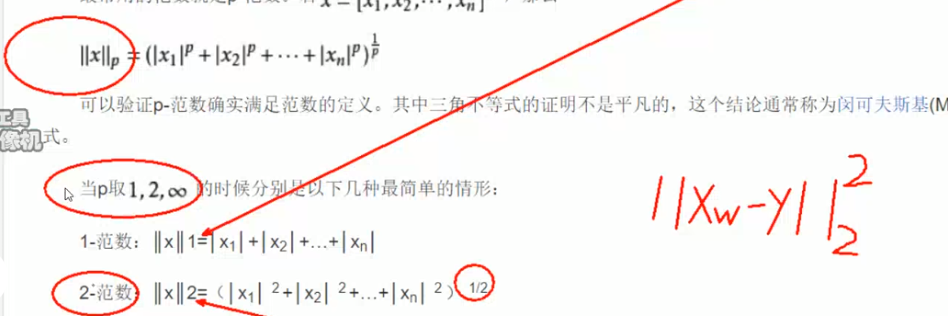# 岭回归

Ridge回归通过对系数的大小进行惩罚来解决普通最小二乘的一些问题 。脊系数使受惩罚的残差平方和最小化：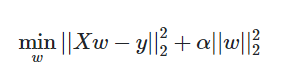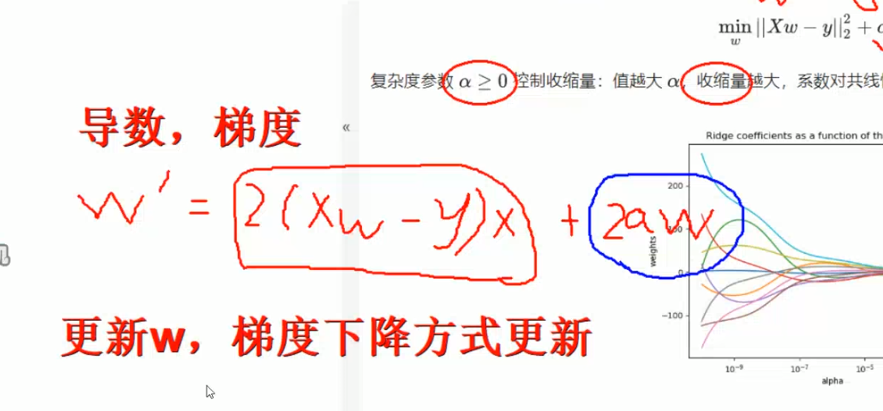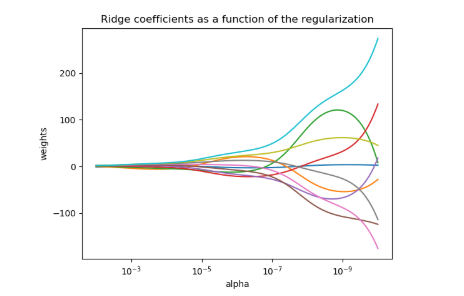# 正则化：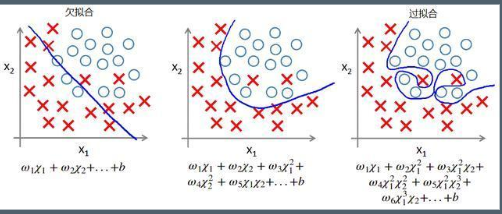# L1正则化

L1正则化，即原损失函数 + 所有权重的平均绝对值 * λ ，其中λ >0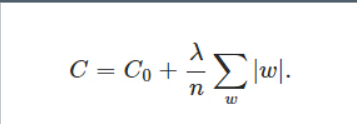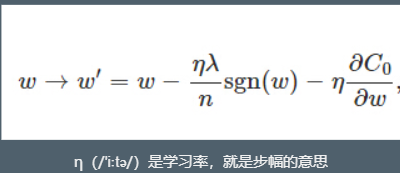# L2正则化

L2正则化，即原损失函数 + 所有权重平方和的平均值 * λ / 2 , λ>0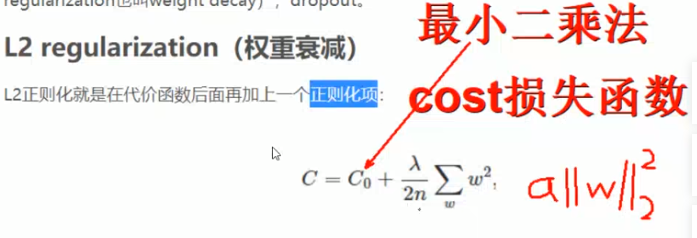# 套索回归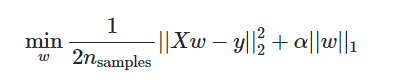minw12nsamples||Xw−y||22+α||w||1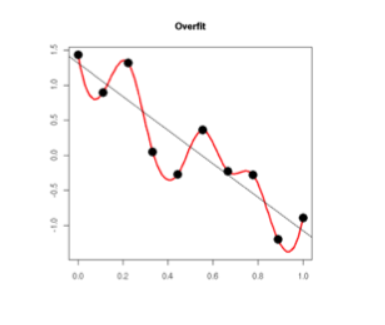# 弹性网络

弹性网络 是一种使用 L1， L2 范数作为先验正则项训练的线性回归模型。 这种组合允许拟合到一个只有少量参数是非零稀疏的模型，就像 Lasso 一样，但是它仍然保持了一些类似于 Ridge 的正则性质。我们可利用 l1_ratio 参数控制 L1 和 L2 的凸组合。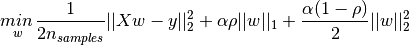# 多任务套索

MultiTaskLasso是，估计多个回归问题共同稀疏系数的线性模型：y是形状的2D阵列。约束条件是所有回归问题（也称为任务）的所选特征都相同。(n_samples, n_tasks)下图比较了通过简单套索或MultiTaskLasso获得的系数矩阵W中非零项的位置。套索估计产生分散的非零值，而MultiTaskLasso的非零值是完整的列。

# 其他回归模型代码演示：

## 导包

import numpy as np

import matplotlib.pyplot as plt
%matplotlib inline

from sklearn.model_selection import train_test_split

#均方误差
from sklearn.metrics import mean_squared_error,r2_score
from sklearn import datasets

# CV crosss validation ：交叉验证
from sklearn.linear_model import LinearRegression,Ridge,Lasso,ElasticNet,ElasticNetCV,LassoCV

## 加载糖尿病数据

diabetes = datasets.load_diabetes()
X = diabetes['data']
y = diabetes['target']

## 训练

X_train,X_test,y_train,y_test = train_test_split(X,y,test_size = 0.15)


## 线性模型

lr = LinearRegression()

lr.fit(X_train,y_train)

# 回归问题的得分，不是准确率
lr.score(X_test,y_test)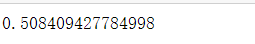## 回归问题得分计算规则

'''The coefficient R^2 is defined as (1 - u/v), where u is the residual
sum of squares ((y_true - y_pred) ** 2).sum() and v is the total
sum of squares ((y_true - y_true.mean()) ** 2).sum().'''

u = ((y_test - y_)**2).sum()
v = ((y_test - y_test.mean())**2).sum()
r2 = 1 - u/v
r2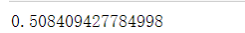score越大，算法越好。

mean_squared_error(y_test,y_)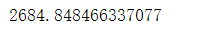# 使用岭回归

rigde = Ridge(alpha=0.001)#alpha越小越不好，当为0的时候，就是线性回归。

rigde.fit(X_train,y_train)

print(rigde.score(X_test,y_test))

y_ = rigde.predict(X_test)

mean_squared_error(y_test,y_)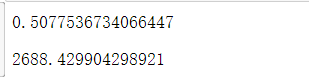## 交叉验证

from sklearn.linear_model import RidgeCV

ridgeCV = RidgeCV(alphas=np.logspace(-5,1,50),scoring='r2',cv = 6)#等比数列找最佳参数

ridgeCV.fit(X_train,y_train)

y_ = ridgeCV.predict(X_test)
r2_score(y_test,y_)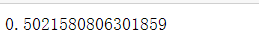ridgeCV = RidgeCV(alphas=np.linspace(0.01,5,50),scoring='r2',cv = 6)#等差数列

ridgeCV.fit(X_train,y_train)

y_ = ridgeCV.predict(X_test)
r2_score(y_test,y_)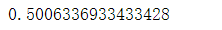#### 机器学习算法实战——线性回归算法

03-31#### 机器学习算法基础

12-18

08-158万+

#### 机器学习总结(一)：线性回归、岭回归、Lasso回归

11-112万+

#### 最小二乘法与岭回归的介绍与对比

06-09663

#### 回归Regression(一元线性回归、岭回归、多元线性回归、多项式回归)

08-26510

#### 机器学习：线性回归、岭回归、Lasso回归

10-312617

#### 线性回归、局部加权线性回归、岭回归、lasso及逐步线性回归

09-01476

#### 线性回归和岭回归

03-22541

#### 线性回归与非线性回归：1.8岭回归 及实战

05-08230

#### 线性回归预测波士顿房价

10-13342

#### 一文读懂线性回归、岭回归和Lasso回归

01-07308

#### 深入理解线性回归与岭回归(数学推导)

08-183828

#### Python高级--线性回归、岭回归（岭际线）、lasso回归

03-106135

#### 机器学习之普通线性回归、岭回归与lasso回归比较

12-1742

#### 机器学习——回归问题（线性回归，岭回归，逐步回归）

10-1690

#### 【机器学习】一文读懂线性回归、岭回归和Lasso回归©️2020 CSDN 皮肤主题: 大白 设计师: CSDN官方博客点击重新获取扫码支付1.余额是钱包充值的虚拟货币，按照1:1的比例进行支付金额的抵扣。
2.余额无法直接购买下载，可以购买VIP、C币套餐、付费专栏及课程。余额充值